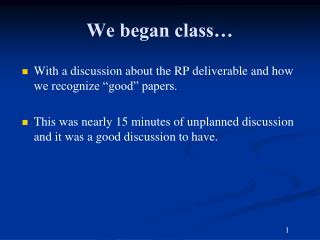DownloadDownload PresentationWe began class…

# We began class…

Télécharger la présentation## We began class…

- - - - - - - - - - - - - - - - - - - - - - - - - - - E N D - - - - - - - - - - - - - - - - - - - - - - - - - - -
##### Presentation Transcript

1. We began class… • With a discussion about the RP deliverable and how we recognize “good” papers. • This was nearly 15 minutes of unplanned discussion and it was a good discussion to have.

2. Review - What is a logic? • A formal language • Syntax – what expressions are legal • Semantics – what legal expressions mean

3. Propositional Logic - Basics • A statement (or logical expression) in propositional logic is similar to a sentenceused in the English language • It is a sentence that can be categorized with a truth value of true or false • Lower-case letters from the middle of the alphabet such as p, q, and r are used to denote propositional logic variables • These variables are the primitives, or basic building blocks in this logic

4. Basics / cont. Table 5.2: Compound expressions formed by using logical connectives

5. Propositional logic: syntax

6. What is a logic? • A formal language • Syntax – what expressions are legal • Semantics – what legal expressions mean • Proof system – a way of manipulating syntactic expressions to get other syntactic expressions (which will tell us something new)

7. Proof methods

8. Truth Tables • In a truth table, F is used to denote false and T is used to denote true, sometimes 0 and 1, respectively • and – true only when both variables are true • or – true when one or both variables are true • p \/ q (p or q) is false only when both p and q are false • XOR (exclusive or)– true when either variable is true, but false when both variables are true

9. Truth Tables / cont. • Unlike the and, or, and exclusive or functions, the not function requires only one variable • not false is true and not true is false • ~p (not p) • implication (⇒) • p ⇒ q (“p implies q” or “if p then q”) • If p is true, then q will result (ex. “If it rains, then the streets will be wet”)

10. Truth Tables / cont. • biconditional(⇔) • p ⇔ q (“p if and only if q” or “p iff q”) • p ⇔ q is true whenever both p and q have the same truth value • i.e., both are true or both are false • Since both variables need to have the same truth value for the biconditional to be true, the biconditional operator is sometimes called the equivalence operator

11. Truth Tables / cont. • The left side of an implication is referred to as the antecedent • The right side of an implication is referred to as the consequent • The converse of an implication is formed by reversing the antecedent and the consequent • The converseof p ⇒ q is q ⇒ p

12. Truth Tables /cont. • The inverse of an implication is formed by negating both the antecedent and consequent • The inverse of p ⇒ q is ~p ⇒ ~q • A useful proof technique in mathematics is a proof by contrapositive • The contrapositive is formed by taking the inverse of the converse of an implication • The contrapositive of p ⇒ q is ~q ⇒ ~p

13. Truth Tables / cont. Table 5.6: Truth table for an implication (column 3) as well as its converse (column 4), inverse (column 5), and contrapositive (column 6), where columns are numbered for ease of reference.

14. Truth Tables /cont. • The symbol ≡ is used to denote that two logical expressions are equivalent by definition (for example, (p ⇒ q) ≡ ~p \/ q) • Such a compound expression is referred to as a tautology or a theorem Table 5.7: Two tautologies in the propositional logic. Observe that in the last two columns, all the entries are true.

15. Truth Tables / cont. • Using a truth table to demonstrate that a logical expression is a tautology and is therefore always true is referred to as a proof by perfect induction • As shown in Table 5.7 (~p \/ ~q) and ~(p /\ q) are always identical in truth value • This theorem is one form of De Morgan’s law • Table 5.8 in the textbook lists additional theorems in propositional logic

16. Propositional inference: enumeration method

17. Enumeration: Solution